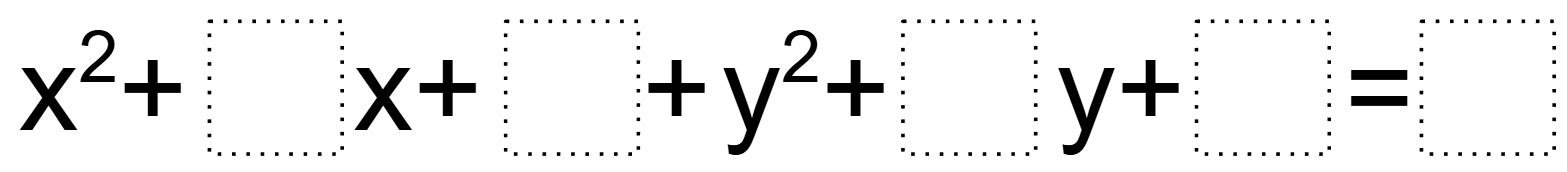# Equation of the Biggest Circle

Directions:

Fill in the blanks with integers (including negatives) that meet the following requirements:

1. The equation’s graph is a circle.
2. The circle has the biggest area
3. The circle is completely inside the first quadrant
4. The circle’s radius is a whole number 1 through 9.### Hint

When graphing an equation of a circle, which form of the equation is the most helpful?

Source: Nanette Johnson

## Pythagorean Inequality

Directions: Use the whole numbers 1 through 6, at most one time each, to find …

### One comment

1.I’m not sure I understand this one. By completely inside, are you saying it cannot touch the axis and are you not allowed to use numbers twice?